# Differential Abundance Analysis

This tool performs a generalized linear model differential abundance test on samples, or groups of samples defined by metadata. The tool models each feature (e.g., an OTU, an organism or species name or a GO term) as a separate Generalized Linear Model (GLM), where it is assumed that abundances follow a Negative Binomial distribution. The Wald test is used to determine significance between group pairs, whereas a Likelihood Ratio test is used in the Across groups (ANOVA-like) comparison. The underlying statistical model is the same as the one used by the Differential Expression for RNA-Seq tool described in details here: http://resources.qiagenbioinformatics.com/manuals/clcgenomicsworkbench/current/index.php?manual=Differential_Expression.html.

To run the tool:

Metagenomics (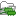) | Abundance Analysis (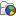) | Differential Abundance Analysis (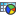)

Select an abundance table with more than one sample as input (i.e., an OTU table table, or a merged functional or profiling table), and if you want to test differential abundance based on metadata defined groups of samples (figure 7.10). It is also possible to correct the results based on a metadata defined group of samples. Finally you can choose whether you want the comparison to be done across groups or between all group pairs.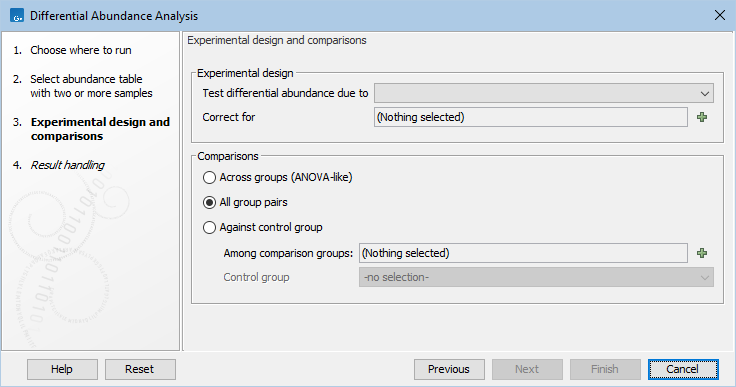Figure 7.10: Specify an abundance table and other parameters.

The tool generates a Venn diagram for three pairwise comparisons at a time (figure 7.11). You can select which comparisons should be shown using the drop down menus in the side panel. Clicking a circle segment in the Venn diagram will select the samples of this segment in the differential abundance analysis table view. The table summarizes abundances, fold changes, differential abundance p-values, multi-sample corrected p-values, etc.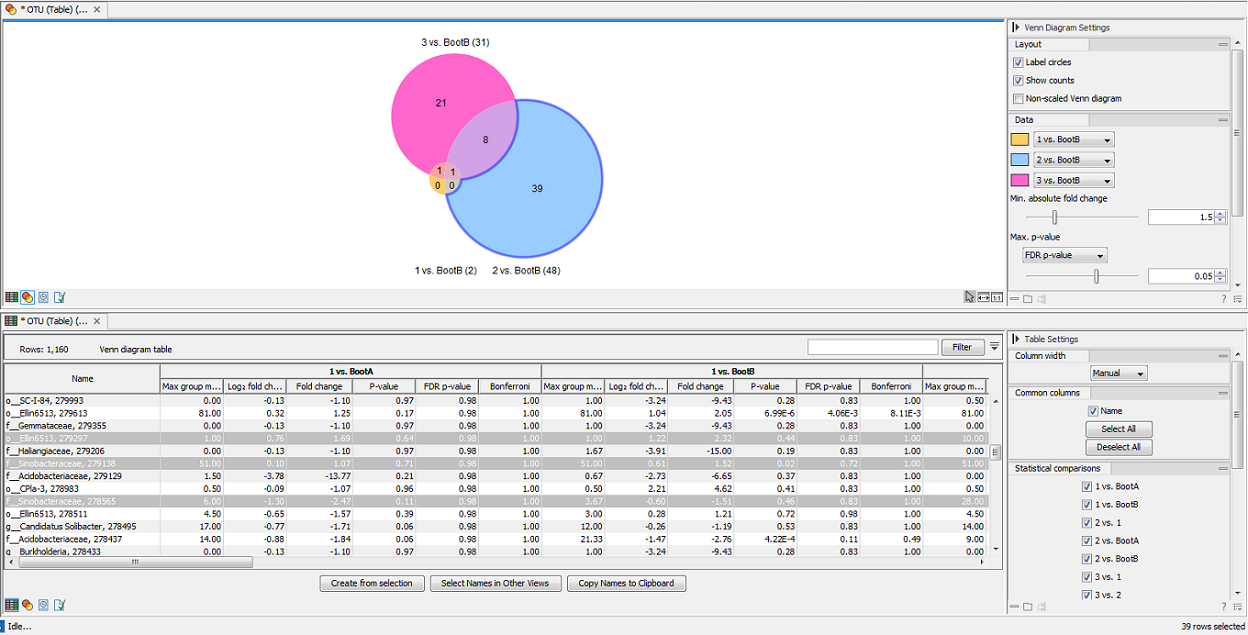Figure 7.11: Venn diagram for three comparisons at a time. Selecting a segment will highlight the samples in the differential abundance table opened below in split view.

The values included in the table for each pairwise comparison are:

• Max group means For each group in the statistical comparison, the average RPKM is calculated. This value is the maximum of the average RPKM's.
• -log 2 The logarithmic fold change.
• Fold change The (signed) fold change. Genes/transcripts that are not observed in any sample have undefined fold changes and are reported as NaN (not a number). Note: Fold changes are calculated from the GLM, which corrects for differences in library size between the samples and the effects of confounding factors. It is therefore not possible to derive these fold changes from the original counts by simple algebraic calculations.
• P-value Standard p-value. Genes/transcripts that are not observed in any sample have undefined p-values and are reported as NaN (not a number).
• FDR p-value The false discovery rate corrected p-value.
• Bonferroni The Bonferroni corrected p-value.

It is possible to create a subset list of samples using the Create from selection button. As usual, the table can be adjusted with the right hand side panel options: it is possible to adjust the column layout, and select which columns should be included in the table.